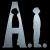## 初学者提问：有关二叉树的中序遍历非递归算法的C语言实现

hwqyc 发布于 2012/09/07 16:23

#include<stdio.h>
#include<malloc.h>
#define NUM_NODE 12
typedef struct Node{
int data;
struct Node *lchild,*rchild;
}BiTreeNode,*BiTree;

BiTree CreateTree(int n){
BiTree bt;
if(n<=0||n>NUM_NODE)return NULL;
if(!(bt=(BiTree)malloc(sizeof(BiTreeNode))))return NULL;
bt->data=n;
printf("%d\t",bt->data);
bt->lchild=CreateTree(2*n);
bt->rchild=CreateTree(2*n+1);
return bt;
}

void FreeTree(BiTree bt){
if(bt){
if(bt->lchild)
FreeTree(bt->lchild);
if(bt->rchild)
FreeTree(bt->rchild);
printf("%d\t",bt->data);
free(bt);
}
}

//中序遍历非递归
void InOrder(BiTree bt){
BiTree *stack,*top,p;
//创建栈
if(!(stack=(BiTree*)malloc(NUM_NODE*sizeof(BiTreeNode)))){
printf("InOrder failer for memory\n");
return;
}
//初始化堆栈
top=stack;
p=bt;
while(p||top>stack){ //p不为NULL或者堆栈不空    为什么不是*top>*stack????
if(p){
*top++=p; //p入栈     为什么不是(*top)++???
p=p->lchild;
}
else{
p=*--top; //栈顶元素出栈，并用p指向     为什么不是p=--(*top)???
if(p) printf("%d\t",p->data);
p=p->rchild;
}
}
}

int main(){
BiTree root;
printf("Create Tree\n");
root=CreateTree(1);
printf("\nInOrder\n");
InOrder(root);
printf("\nFree Tree\n");
FreeTree(root);
return 0;
}

0top 和 stack 是栈的内存地址。 而*top 和 *stack 则是栈里的元素的内容。h